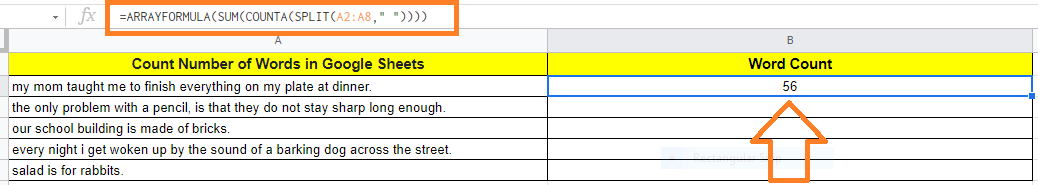# How to Count the Number of Words in Google Sheets (2 Easy Formulas)

When you are working in Google Docs, you can easily count the number of words for the given dataset with the help of an in-built function. When it comes to Google Sheets, we don’t have any in-built function to count the number of words. However, if you are working on Google Spreadsheet and want to count the number of words for the given dataset, then you can use the LEN and SUBSTITUTE datasets. In this article, let’s understand how to get the word count with the help of the LEN and SUBSTITUTE function with the help of Google Sheet tips provided on this page. Read on to find out more.

## How to Count the Number of Words in Google Sheets?

Follow the steps as listed below to get the word count in Google Sheet:

• Step 1: Select the cell where you would like to get the word count.
• Step 2: Now enter the formula as “=LEN(A1)LEN(SUBSTITUTE(A1,” “,“”))+1“.
• Step 3: You will see the results. Now the drag the Fill Handle from the formula applied cell to another cell to get the word count for other cells as well.

You will get the word count for the text you have entered as shown below.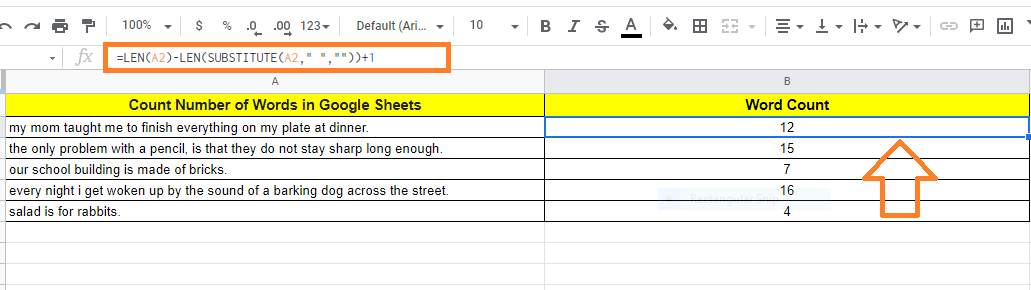Alternatively, you can also use the formula “=IF(A2=””,””,COUNTA(SPLIT(A2,” “)))” to count the number of words in the given dataset.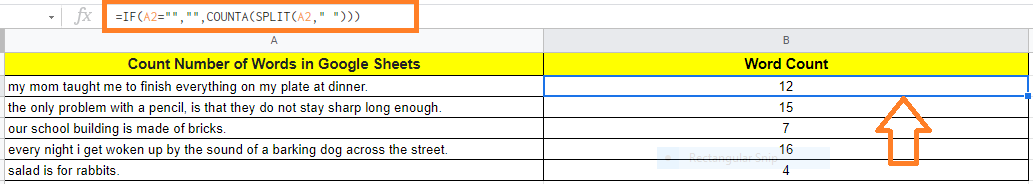### How to Count the Number of Words with Extra Spaces in-between Text?

In some instances, we might have 2 or more extra spaces in between the sentence. In those cases, if we use the above formulas, then the results drawn would not be accurate. Thus to overcome this we can use the TRIM formula along with LEN and SUBSTITUTE functions. Follow the steps listed below to count the number of words in a sentence that has extra spaces.

• Step 1: Move the cell where you would like to get the word count of a sentence which has extra space.
• Step 2: Now enter the formula as “=LEN(TRIM(A1))-LEN(SUBSTITUTE(TRIM(A1),” “,””))+1“.
• Step 3: Press the “Enter” button. You will see the results.

To apply the same formula all over the cells, you can drag the fill handle from the formula applied cell.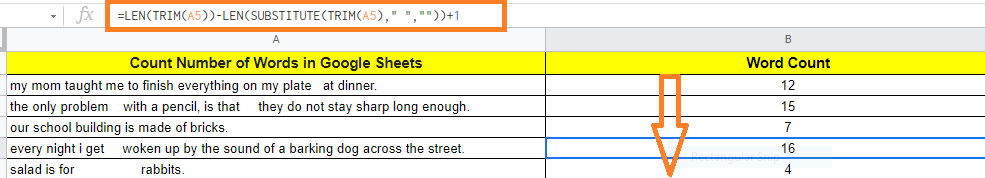### Working of LEN & SUBSTITUTE Formula

• The function’s LEN(A1) section returns the total number of characters in the text string (including the spaces).
• The SUBSTITUTE function is used in the LEN(SUBSTITUTE(A1,” “,“”) portion to remove all the spaces. The length of the characters in the text string is then calculated.
• The total number of words is calculated by adding the value 1 to the difference between these two formulas.

### How to Count Specific Word in Google Sheets?

Let’s consider you have a dataset where you want to count the specific word occurrence. For example, if you have the following dataset and want to count the occurrence of the word “THE” in the dataset.

Let’s understand how to do this by following the steps listed below:

• Step 1: Move the cell where you would like to count the specific word.
• Step 2: Now enter the formula “=IF(A2=””,””,COUNTIF(SPLIT(A2,” “),”the”))“.
• Step 3: Press the “Enter” button. You will see the results.
• Step 4: Drag the fill handle from the formula applied cell to apply the same formula across other cells.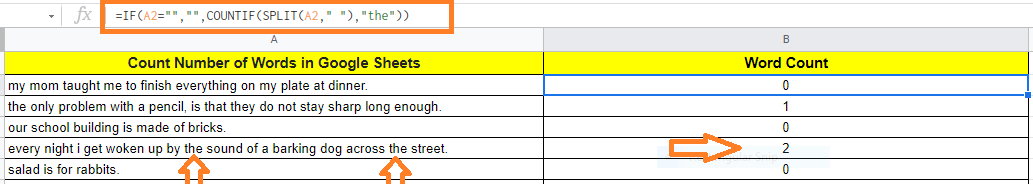### Tips to Count Number of Words in Google Sheets

• You may also rapidly calculate the word count using Google Docs. To acquire the word and character count, just copy and paste the text into any blank Google Docs document and hit Control + Shift + C (all at once).
• Word Count for Entire Column: To get the word count for the entire column, you can simply use the formula “=ARRAYFORMULA(SUM(COUNTA(SPLIT(A2:A8,” “))))”. Here we have chosen A2:A8 since it’s the data range. Based on the cell range, you can change this formula.